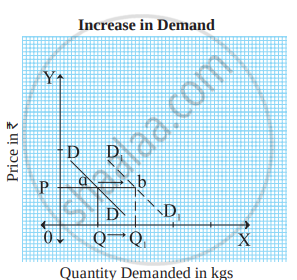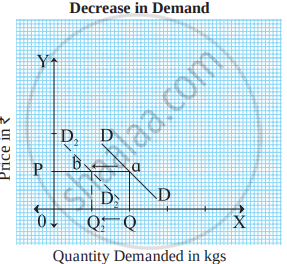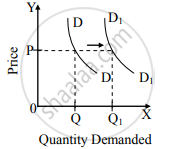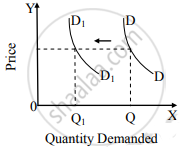# Change in Demand

## Notes

#### Changes in Demand :

When demand for a commodity increases or decreases due to changes in other factors and price remains constant, it is known as changes in demand. It is of two types :

#### 1) Increase in demand :

It refers to an increase in quantity demanded due to favourable changes in other factors like tastes, the income of the consumer, climatic conditions etc. and price remains constant.  Demand curve shifts to the right-hand side of the original demand curve. This can be explained with the help of a figure.As shown in figure, DD is the original demand curve. Demand curve shifts outward to the right from DD to D1 D1 which indicates increase in demand.

2) Decrease in demand :

It refers to decrease in quantity demanded due to unfavourable changes in other factors like tastes, income of the consumer, climatic conditions etc. and price remains constant.  Demand curve shifts to left hand side of the original demand curve. This can be explained with the help of figureAs shown in figure, DD is the original demand curve. Demand curve shifts outward to the right from DD to D1 D1 which indicates increase in demand.

### For Better Understanding:

 Increase in Demand Decrease in Demand When more quantity is demanded than before at the same price, it is called an increase in demand. When less quantity is demanded than before at the same price, it is called a decrease in demand. Increase in demand takes place due to favourable changes in factors other than price like fashion, income, taxation policy, advertisements, etc. Decrease in demand takes place due to unfavourable changes in factors other than price like fashion, income, taxation policy, advertisements, etc. An increase in demand is indicated by a shift in the demand curve to the right. A decrease in demand is indicated by a shift in the demand curve to left. When there is an increase in demand, the demand curve shifts to the right from DD to D1​D2​ as shown in the figure.When there is a decrease in demand the demand curve shift to the left from DD to D2​D2​ as shown in the figure.If you would like to contribute notes or other learning material, please submit them using the button below.

### Shaalaa.com

DEMAND ANALYSIS Part 5 (Change in Demand) [00:09:28]
S
0%